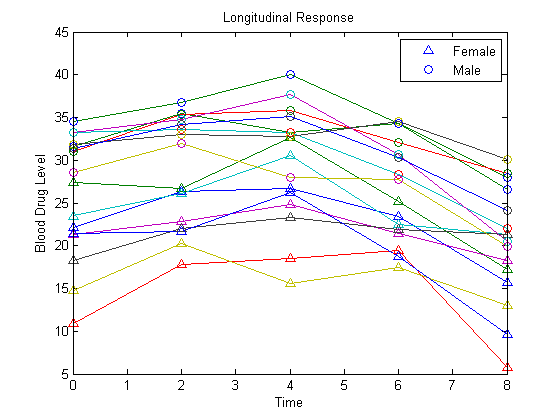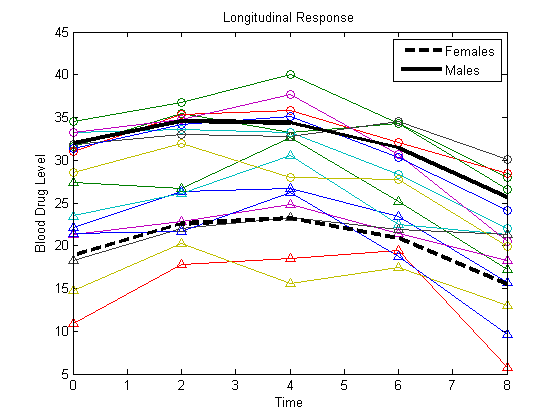## Longitudinal Analysis

This example shows how to perform longitudinal analysis using `mvregress`.

`load longitudinalData`

The matrix `Y` contains response data for 16 individuals. The response is the blood level of a drug measured at five time points (t = 0, 2, 4, 6, and 8). Each row of `Y` corresponds to an individual, and each column corresponds to a time point. The first eight subjects are female, and the second eight subjects are male. This is simulated data.

### Plot data.

Plot the data for all 16 subjects.

```figure() t = [0,2,4,6,8]; plot(t,Y) hold on hf = plot(t,Y(1:8,:),'^'); hm = plot(t,Y(9:16,:),'o'); legend([hf(1),hm(1)],'Female','Male','Location','NorthEast') title('Longitudinal Response') ylabel('Blood Drug Level') xlabel('Time') hold off```### Define design matrices.

Let yij denote the response for individual i = 1,...,n measured at times tij, j = 1,...,d. In this example, n = 16 and d = 5. Let Gi denote the gender of individual i, where Gi = 1 for males and 0 for females.

Consider fitting a quadratic longitudinal model, with a separate slope and intercept for each gender,

`${y}_{ij}={\beta }_{0}+{\beta }_{1}{G}_{i}+{\beta }_{2}{t}_{ij}+{\beta }_{3}{t}_{ij}^{2}+{\beta }_{4}{G}_{i}×{t}_{ij}+{\beta }_{5}{G}_{i}×{t}_{ij}^{2}+{\epsilon }_{ij},$`

where ${\epsilon }_{i}=\left({\epsilon }_{i1},\dots ,{\epsilon }_{id}{\right)}^{\prime }\sim MVN\left(0,\Sigma \right)$. The error correlation accounts for clustering within an individual.

To fit this model using `mvregress`, the response data should be in an n-by-d matrix. `Y` is already in the proper format.

Next, create a length-n cell array of d-by-K design matrices. For this model, there are K = 6 parameters.

For individual i, the 5-by-6 design matrix is

`$X\left\{i\right\}=\left(\begin{array}{cccccc}1& {G}_{i}& {t}_{i1}& {t}_{i1}^{2}& {G}_{i}×{t}_{i1}& {G}_{i}×{t}_{i1}^{2}\\ 1& {G}_{i}& {t}_{i2}& {t}_{i2}^{2}& {G}_{i}×{t}_{i2}& {G}_{i}×{t}_{i2}^{2}\\ ⋮& ⋮& ⋮& ⋮& ⋮& ⋮\\ 1& {G}_{i}& {t}_{i5}& {t}_{i5}^{2}& {G}_{i}×{t}_{i5}& {G}_{i}×{t}_{i5}^{2}\end{array}\right),$`

corresponding to the parameter vector

`$\beta =\left(\begin{array}{l}{\beta }_{0}\\ {\beta }_{1}\\ \text{\hspace{0.17em}}\text{\hspace{0.17em}}\text{\hspace{0.17em}}⋮\\ {\beta }_{5}\end{array}\right).$`

The matrix `X1` has the design matrix for a female, and `X2` has the design matrix for a male.

Create a cell array of design matrices. The first eight individuals are females, and the second eight are males.

```X = cell(8,1); X(1:8) = {X1}; X(9:16) = {X2}; ```

### Fit the model.

Fit the model using maximum likelihood estimation. Display the estimated coefficients and standard errors.

```[b,sig,E,V,loglikF] = mvregress(X,Y); [b sqrt(diag(V))]```
```ans = 18.8619 0.7432 13.0942 1.0511 2.5968 0.2845 -0.3771 0.0398 -0.5929 0.4023 0.0290 0.0563```

The coefficients on the interaction terms (in the last two rows of `b`) do not appear significant. You can use the value of the loglikelihood objective function for this fit, `loglikF`, to compare this model to one without the interaction terms using a likelihood ratio test.

### Plot fitted model.

Plot the fitted lines for females and males.

```Yhatf = X1*b; Yhatm = X2*b; figure() plot(t,Y) hold on plot(t,Y(1:8,:),'^',t,Y(9:16,:),'o') hf = plot(t,Yhatf,'k--','LineWidth',3); hm = plot(t,Yhatm,'k','LineWidth',3); legend([hf,hm],'Females','Males','Location','NorthEast') title('Longitudinal Response') ylabel('Blood Drug Level') xlabel('Time') hold off```### Define a reduced model.

Fit the model without interaction terms,

`${y}_{ij}={\beta }_{0}+{\beta }_{1}{G}_{i}+{\beta }_{2}{t}_{ij}+{\beta }_{3}{t}_{ij}^{2}+{\epsilon }_{ij},$`

where ${\epsilon }_{i}=\left({\epsilon }_{i1},\dots ,{\epsilon }_{id}{\right)}^{\prime }\sim MVN\left(0,\Sigma \right)$.

This model has four coefficients, which correspond to the first four columns of the design matrices `X1` and `X2` (for females and males, respectively).

```X1R = X1(:,1:4); X2R = X2(:,1:4); XR = cell(8,1); XR(1:8) = {X1R}; XR(9:16) = {X2R}; ```

### Fit the reduced model.

Fit this model using maximum likelihood estimation. Display the estimated coefficients and their standard errors.

```[bR,sigR,ER,VR,loglikR] = mvregress(XR,Y); [bR,sqrt(diag(VR))]```
```ans = 19.3765 0.6898 12.0936 0.8591 2.2919 0.2139 -0.3623 0.0283```

### Conduct a likelihood ratio test.

Compare the two models using a likelihood ratio test. The null hypothesis is that the reduced model is sufficient. The alternative is that the reduced model is inadequate (compared to the full model with the interaction terms).

The likelihood ratio test statistic is compared to a chi-squared distribution with two degrees of freedom (for the two coefficients being dropped).

```LR = 2*(loglikF-loglikR); pval = 1 - chi2cdf(LR,2)```
```pval = 0.0803```
The p-value `0.0803` indicates that the null hypothesis is not rejected at the 5% significance level. Therefore, there is insufficient evidence that the extra terms improve the fit.